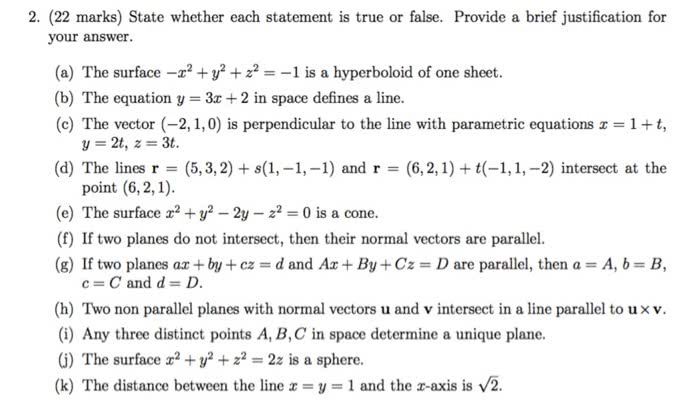1
0
watching
120
views

#2. (22 marks) State whether each statement is true or false. Provide a brief justification for your answer. (a) The surface-z2 + y2 Ï2--l is a hyperboloid of one sheet. (b) The equation y = 3x + 2 in space defines a line. (c) The vector (-2,1,0) is perpendicular to the line with parametric equations x = 1 + t, y-2t, z = 3t. (d) The lines r = (5,3,2)+ s(1,-1,-1) and r = (6,2, 1)+1(-1,1,-2) intersect at the point (6, 2,1) (e) The surface x2 + y2-2y-22 = 0 is a cone. (f) If two planes do not intersect, then their normal vectors are parallel. (g) If two planes ax + by + cz = d and Ax + By + Cz = D are parallel, then a = A, b = B, c=Cand d = D. (h) Two non parallel planes with normal vectors u and v intersect in a line parallel to uxv (i) Any three distinct points A, B,C in space determine a unique plane. (G) The surface2 +2-2z is a sphere. (k) The distance between the line x = y = 1 and the x-axis is V2. Show transcribed image text 2. (22 marks) State whether each statement is true or false. Provide a brief justification for your answer. (a) The surface-z2 + y2 Ï2--l is a hyperboloid of one sheet. (b) The equation y = 3x + 2 in space defines a line. (c) The vector (-2,1,0) is perpendicular to the line with parametric equations x = 1 + t, y-2t, z = 3t. (d) The lines r = (5,3,2)+ s(1,-1,-1) and r = (6,2, 1)+1(-1,1,-2) intersect at the point (6, 2,1) (e) The surface x2 + y2-2y-22 = 0 is a cone. (f) If two planes do not intersect, then their normal vectors are parallel. (g) If two planes ax + by + cz = d and Ax + By + Cz = D are parallel, then a = A, b = B, c=Cand d = D. (h) Two non parallel planes with normal vectors u and v intersect in a line parallel to uxv (i) Any three distinct points A, B,C in space determine a unique plane. (G) The surface2 +2-2z is a sphere. (k) The distance between the line x = y = 1 and the x-axis is V2.